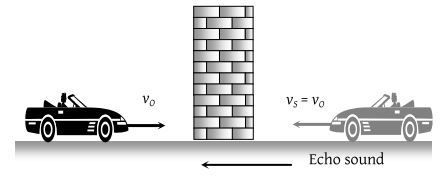A motor car blowing a horn of frequency 124vib/sec moves with a velocity 72 km/hr towards a tall wall. The frequency of the reflected sound heard by the driver will be (velocity of sound in air is 330 m/s)

(1) 109 vib/sec

(2) 132 vib/sec

(3) 140 vib/sec

(4) 248 vib/sec

(3) Similar to previous questionThe frequency of reflected sound heard by the driver

${n}^{\text{'}}=n \left(\frac{v-\left(-{v}_{0}\right)}{v-{v}_{s}}\right)=n \left(\frac{v+{v}_{0}}{v-{v}_{s}}\right)$

$=124\left[\frac{330+\left(72×5/18\right)}{330-\left(72×5/18\right)} \right]$= 140 vibration/sec.

Difficulty Level:

• 6%
• 38%
• 58%
• 0%